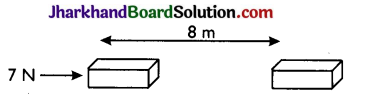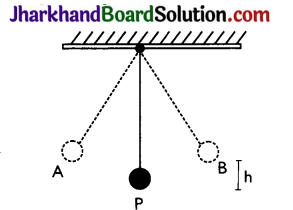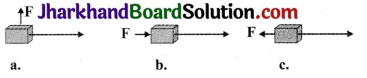# JAC Class 9th Science Solutions Chapter 11 Work and Energy

## JAC Board Class 9th Science Solutions Chapter 11 Work and Energy

JAC Class 9th Science Work and Energy InText Questions and Answers

Page 148

Question 1.
A force of 7N acts on an object. The displacement is, say 8m, in the direction of the force. Let us take it that the force acts on the object through the displacement. What is the work done in this case?
When a force F acts on an object to displace it through a distance s in its direction, the work done, W on the body by the force is given by:
Work done = Force × Displacement
W = F × s
Where, F = 7N, and s = 8m Therefore, work done,
W = 7 × 8 = 56 Nm = 56JPage 149

Question 1.
When do we say that work is done?
Work is said to be done whenever the following two conditions are satisfied:

1. A force acts on the body.
2. There is a displacement in the body caused by the applied force along the direction of the force.

Question 2.
Write an expression for the work done when a force is acting on an object in the direction of its displacement.
When a force ‘F’ displaces a body through a distance ‘s’ in the direction of the applied force, the work done, ‘W’ on the body is given by the expression:
Work done = Force × Displacement W = F × sQuestion 3.
Define 1J of work.
1J is the amount of work done by a force of 1N on an object that displaces it through a distance of 1m in the direction of the applied force.

Question 4.
A pair of bullocks exerts a force of 140N on the plough. The field being ploughed is 15m long. How much work is done in ploughing the length of the field?
Work done by the bullocks is given by the expression:
Work done = Force × Displacement
W=F × s
Where,
Applied force, F = 140N
Displacement, s = 15 m
W= 140 × 15 = 2100J
Hence, 2100 J of work is done in ploughing the length of the field.

Page 152

Question 1.
What is the kinetic energy of an object?
The energy possessed by a body by the virtue of its motion is called kinetic energy. It has various applications:
(a) Kinetic energy of flowing water is used to generate electricity.
(b) Kinetic energy of hammer is used in driving a nail into a log of wood.
(c) Kinetic energy of air is used to run windmills, etc.

Question 2.
Write an expression for the kinetic energy of an object.
If a body of mass ‘m’ is moving with a velocity ‘v’ its kinetic energy K.E. is given by the expression,
K.E. = $$\frac{1}{2}$$ mv2
Its SI unit is joule (J).

Question 3.
The kinetic energy of an object of mass, m moving with a velocity of 5 m s-1 is 25J. What will be its kinetic energy when its velocity is doubled? What will be its kinetic energy when its velocity is increased three times?
K.E. of the object = 25J
Velocity of the object, v = 5 m/s
∴ K.E. = $$\frac{1}{2}$$ mv2
25 = $$\frac{1}{2}$$m(5)2
m = 2 kg

(a) If velocity is doubled, v = 10m/s, m = 2 kg
K.E. = $$\frac{1}{2}$$ mv2 = $$\frac{1}{2}$$(2) × (10)2 = 100J.
K.E will become four times.

(b) If velocity is increased 3 times,
v = 15 m/s, m = 2 kg
K.E = $$\frac{1}{2}$$ mv2 = $$\frac{1}{2}$$ × 2 × (15)2 = 225J.
K.E will become nine times.

Page 156

Question 1.
What is power?
Power is the rate of doing work or the rate of transfer of energy. If W is the amount of work done in time t, then power is given by the expression,
Power = $$\frac{Work}{Time }$$ = $$\frac{w}{t}$$
It is expressed in watt (W).
watt = $$\frac{Joule}{Second}$$

Question 2.
Define 1 watt of power.
A body is said to have a power of 1 watt if it does 1 joule work in 1 s, i.e.,
1W =$$\frac{1 \mathrm{~J}}{1 \mathrm{~s}}$$

Question 3.
A lamp consumes 1000J of electrical energy in 10s. What is its power?
Power = $$\frac{Work done}{Time }$$
Work done = Energy consumed by the lamp = 1000J
Time = 10s
Power = $$\frac{1000}{10}$$ = 100J s-1 = 100WQuestion 4.
Define average power.
The average power is defined as the total work done or total energy consumed divided by the total time taken.
Average power = $$\frac{Total work done}{Total time taken }$$

JAC Class 9th Science Work and Energy Textbook Questions and Answers

Question 1.
Look at the activities listed below. Reason out whether or not work is done in the light of your understanding of the term ‘work’.
1. Suma is swimming in a pond.
2. A donkey is carrying a load on its back.
3. A windmill is lifting water from a well.
4. A green plant is carrying out photosynthesis.
5. An engine is pulling a train.
6. Foodgrains are getting dried in the sun.
7. A sailboat is moving due to wind energy.
Work is done whenever the following two conditions are satisfied:

• A force acts on the body.
• There is a displacement of the body by the application of force.
1. While swimming, Suma applies a force to push the water backwards. Therefore, Suma swims in the forward direction under the influence of the forward reaction of water. Here, the force causes a displacement. Hence, work is done by Suma while swimming.
2. While carrying a load, the donkey has to apply a force in the upward direction. But, displacement of the load is in the forward direction. Since, displacement is perpendicular to force, the work done is zero.
3. A windmill works against the gravitational force to lift water. Hence, work is done by the windmill in lifting water from the well.
4. In this case, there is no displacement of the leaves of the plant. Therefore, the work done is zero.
5. An engine applies force to pull the train. This allows the train to move in the direction of force. Therefore, there is a displacement of the train in the direction of force. Hence, work is done by the engine on the train.
6. Foodgrains do not move in the presence of solar energy. Hence, the work done is zero when the foodgrains are getting dried in the sun.
7. Wind energy applies a force on the sailboat to push it in the forward direction. Therefore, there is a displacement in the boat in the direction of force. Hence, work is done by wind on the boat.

Question 2.
An object thrown at a certain angle to the ground moves in a curved path and falls back to the ground. The initial and the final points of the path of the object lie on the same horizontal line. What is the work done by the force of gravity on the object?
Work done by the force of gravity on the object is zero. Force of gravity acts in the vertically downward direction and the distance covered by the object is in the horizontal direction. As there is no  displacement in the direction of force, hence the work done is zero.

Question 3.
A battery lights a bulb Describe the energy changes involved in the process.
When a bulb is connected to a battery, the chemical energy of the battery is transformed into electrical energy. When the bulb receives this electrical energy, it converts it into heat and light energy. Hence, the transformation of energy in the given situation can be shown as:
Chemical Energy → Electrical Energy → Heat Energy + Light Energy

Question 4.
Certain force acting on a 20 kg mass changes its velocity from 5 ms-1 to 2 ms-1. Calculate the work force.
Here,
m = 20 kg,
u = 5 m-1,
v = 2 m-1
Work done = Change in K.E.
W = $$\frac{1}{2}$$ mv2 – $$\frac{1}{2}$$ mu2
= $$\frac{1}{2}$$ × 20 × (22 – 52)
= 10 × (4 – 25)
= – 10 × 21 = – 210J
The negative sign indicates that work is done against the applied force.

Question 5.
A mass of 10 kg is at a point A on a table. It is moved to a point B. If the line joining A and B is horizontal, what is the work done on the object by the gravitational force? Explain your answer.
Work done by gravity depends only on the vertical displacement of the body. It does not depend upon the path of the body. Therefore, work done by gravity is given by the expression,
W = mgh
Where, vertical displacement, h = 0
∴ W = mg × 0 = 0
Hence, the work done by gravity on the body is zero.Question 6.
The potential energy of a freely falling object decreases progressively. Does this violate the law of conservation of energy? Why?
No. The process does not violate the law of conservation of energy. This is because when a body falls from a height, its potential energy progressively changes into kinetic energy. The decrease in potential energy is equal to the increase in kinetic energy of the body. During this process, the total mechanical energy of the body remains conserved. Therefore, the law of conservation of energy is not violated.

Question 7.
What are the various energy transformations that occur when you are riding a bicycle?
While riding a bicycle, the muscular energy of the rider gets transformed into heat energy and kinetic energy of the bicycle. Heat energy heats the rider’s body. Kinetic energy provides velocity to the bicycle. The transformation can be shown as: Muscular Energy → Kinetic Energy + Heat Energy During this transformation, the total energy remains conserved.

Question 8.
Does the transfer of energy take place when you push a huge rock with all your might and fail to move it? Where is the energy you spend going?
When we push a huge rock, there is no transfer of muscular energy to the stationary rock. Also, there is no loss of energy because muscular energy is transferred into heat energy which causes our body to become hot.

Question 9.
A certain household has consumed 250 units of energy during a month. How much energy is this in joules?
1 unit of energy is equal to 1 kilowatt hour (kW h).
1 unit = 1 kW h
1 kWh = 3.6 × 106J
Therefore, 250 units of energy
= 250 × 3.6 × 106 = 9 × 108J

Question 10.
An object of mass 40 kg is raised to a height of 5m above the ground. What is its potential energy? If the object is allowed to fall, find its kinetic energy when it is half – way down.
Gravitational potential energy is given by the expression,
PE= mgh Where,
h = Vertical displacement = 5 m
m = Mass of the object = 40 kg
g = Acceleration due to gravity = 9.8 ms-2
PE = 40 × 5 × 9.8= 1960J.
At half – way down, the potential energy of the object will be $$\frac{1960}{2}$$ = 980J.
At this point, the object has an equal amount of potential and kinetic energy. This is due to the law of conservation of energy. Hence, half – way down, the kinetic energy of the object will also be 980J.

Question 11.
What is the work done by the force of gravity on a satellite moving round the earth? Justify your answer.
Zero. When the satellite moves around the earth, the force of gravity acts on it along the radius of its orbit, while its direction of motion is along the tangent to the orbit at any point. Thus, the force acts perpendicular to the displacement. Hence, the work done on the satellite is zero.

Question 12.
Can there be displacement of an object in the absence of any force acting on it? Think. Discuss this question with your friends and teacher.
Yes. For a uniformly moving object. Suppose an object is moving with a constant velocity. The net force acting on it is zero. But, there is a displacement along the motion of the object. Hence, there can be a displacement without a force.

Question 13.
A person holds a bundle of hay over his head for 30 minutes and gets tired. Has he done some work or not? Justify your answer.
When a person holds a bundle of hay over his head, there is no displacement in the bundle of hay. Although, force of gravity is acting on the bundle, the person is not applying any force on it. Hence, in the absence of force and displacement, work done by the person on the bundle is zero.

Question 14.
An electric heater is rated 1500 W. How much energy does it use in 10 hours?
Ans.
Energy consumed by an electric heater can be obtained with the help of the expression,
P= $$\frac{\mathrm{W}}{\mathrm{t}}$$
Where, power rating of the heater,
P= 1500 W
Time for which the heater has operated, t = 10h
Work done = Energy consumed by the heater
Therefore, energy consumed
= P × t = 1500 W × 10 h
= $$\frac{15000}{1000}$$ = 15kW hQuestion 15.
Illustrate the law of conservation of energy by discussing the energy changes which occur when we draw a pendulum bob to one side and allow it to oscillate. Why does the bob eventually come to rest? What happens to its energy eventually? Is it a violation of the law of conservation of energy?The law of conservation of energy states that energy can neither be created nor destroyed. It can only be converted from one form to another. Consider the case of an oscillating pendulum. When a pendulum moves from its mean position P to either of its extreme positions A or B, it rises through a height ‘h’ above the mean level ‘P’. At this point, the kinetic energy of the bob changes completely into potential energy.

The kinetic energy becomes zero, and the bob possesses only potential energy. As it moves towards point P, its potential energy decreases progressively. Accordingly, the kinetic energy increases. As the bob reaches point P, its potential energy becomes zero and the bob possesses only kinetic energy. This process is repeated as long as the pendulum oscillates. The bob does not oscillate forever. It comes to rest because air resistance resists its motion.

The pendulum loses its kinetic energy to overcome this friction and stops after some time. The law of conservation of energy is not violated because the energy lost by the pendulum to overcome friction is gained by its surroundings. Hence, the total energy of the pendulum and the surrounding system remains conserved.

Question 16.
An object of mass, m is moving with a constant velocity, v. How much work should be done on the object in order to bring the object to rest?
Work done on the object = Change in K.E. of the object.
W = $$\frac{1}{2}$$ mv2 – $$\frac{1}{2}$$ m(0)2 = $$\frac{1}{2}$$ mv2
To bring the object to rest, $$\frac{1}{2}$$ mv2 amount of work is required to be done on the object.

Question 17.
Calculate the work required to be done to stop a car of 1500 kg moving at a velocity of 60 km/h?
Kinetic energy, KE = $$\frac{1}{2}$$ mv2
Where,
mass of the car, m = 1500 kg and velocity of the car,
v = 60 km/h = $$\frac{60 \times 5}{18}$$ ms-1
KE = $$\frac{1}{2}$$ × 1500 × $$\left(\frac{60 \times 5}{18}\right)^{2}$$
= 20.8 × 104 J
Hence, 20.8 × 104 J of work is required to stop the car.

Question 18.
In each of the following, a force, F is acting on an object of mass, m. The direction of displacement is from west to east shown by the longer arrow. Observe the diagrams carefully and state whether the work done by the . force is negative, positive or zero.1. In this case, the direction of force acting on the block is perpendicular to the displacement. Therefore, work done by force on the block will be zero.
2. In this case, the direction of force acting on the block is in the direction of displacement. Therefore, work done by force on the block will be positive.
3. In this case, the direction of force acting on the block is opposite to the direction of displacement. Therefore, work done by the force on the block will be negative.Question 19.
Soni says that the acceleration of an object could be zero even when several forces are acting on it. Do you agree with her? Why?
Acceleration in an object could be zero even when several forces are acting on it. This happens when all the forces cancel out each other, i.e., the net force acting on the object is zero. For a uniformly moving object, the net force acting on the object is zero. Hence, the acceleration of the object is zero. Hence, Soni is right.

Question 20.
Find the energy in kW h consumed in 10 hours by four devices of power 500 W each.
Here, P = 500 W = $$\frac{500}{1000}$$ kW= 0.5 kW and t =10 h